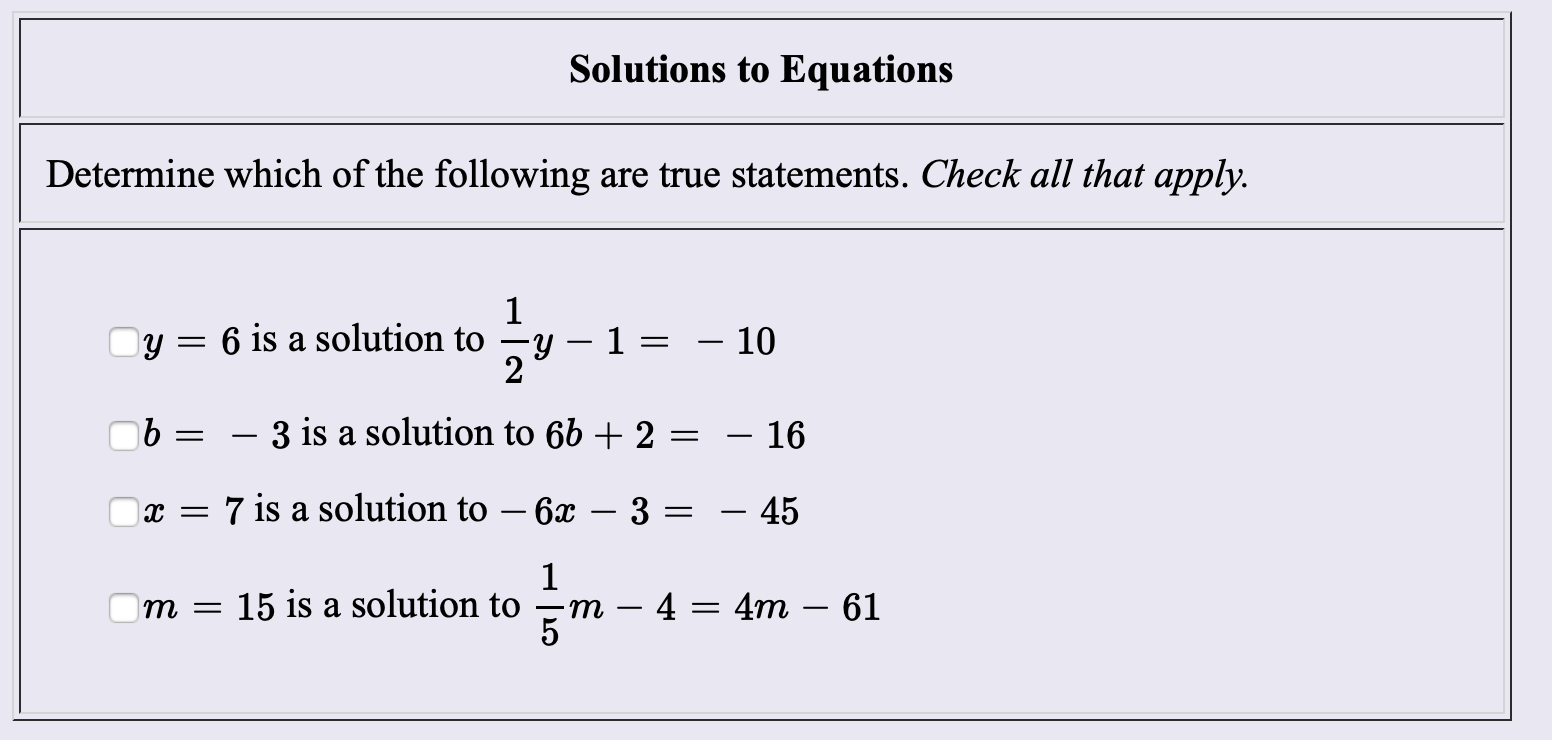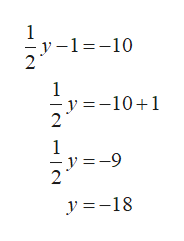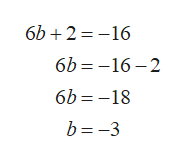Solutions to EquationsDetermine which of the followingare true statements. Check all that apply1y 12- 10Oy= 6 is a solution to- 3 is a solution to 6b + 2- 167 is a solution to - 6x- 453х —14 4m 6115 is a solution toт —т5

Questionhelp_outlineImage TranscriptioncloseSolutions to Equations Determine which of the following are true statements. Check all that apply 1 y 1 2 - 10 Oy= 6 is a solution to - 3 is a solution to 6b + 2 - 16 7 is a solution to - 6x - 45 3 х — 1 4 4m 61 15 is a solution to т — т 5 fullscreen
Step 1

Check which of the statement is true or false.

In 1st statement we have to check: Is y=6 solution of the equation 1/2y-1=-10 or not. Then,help_outlineImage Transcriptionclose1 y-1-10 1 y =-101 2 1 =-9 y =-18 fullscreen
Step 2

Therefore, y=9 is not solution of the linear equation 1/2y-1=-10.

In 2nd statement, we have to check: Is b=-3 the solution of 6b+2=-16 or not. Thenhelp_outlineImage Transcriptionclose6b 216 6b -16 2 6b -18 b -3 fullscreen
Step 3

This shows that b=-3 is the solution of the equation 6b+2=-16.

Now, in the 3rd statement, w...

Want to see the full answer?

See Solution

Want to see this answer and more?

Our solutions are written by experts, many with advanced degrees, and available 24/7

See Solution
Tagged in

Equations and In-equations